# Selina Solutions Concise Mathematics Class 6 Chapter 17 Idea of Speed, Distance and Time

Selina Solutions Concise Mathematics Class 6 Chapter 17 Idea of Speed, Distance and Time contains accurate answers pertaining to speed, distance and time in the given exercise problems. The main intention of providing solutions is to increase analytical and problem solving proficiency among students. Students who aim to obtain expertise in Mathematics are suggested to practice textbook questions, using solutions PDF. For a stronghold on the topics, students can refer to Selina Solutions Concise Mathematics Class 6 Chapter 17 Idea of Speed, Distance and Time PDF, from the links offered below.

Chapter 17 discusses important problems relying on speed, distance and time. Students obtain the technique of solving complex problems with ease, using solutions PDF, designed by experts at BYJU’S.

## Selina Solutions Concise Mathematics Class 6 Chapter 17: Idea of Speed, Distance and Time Download PDF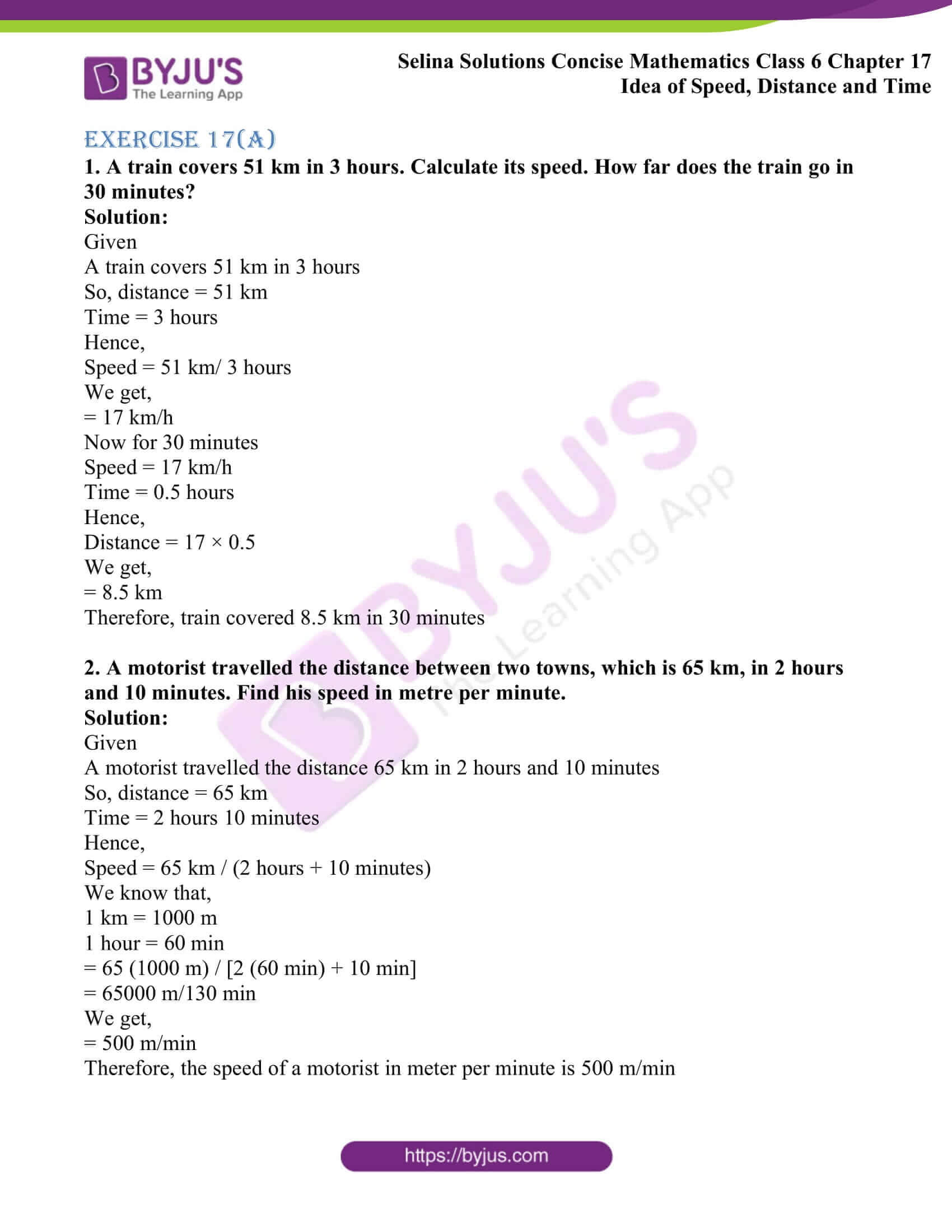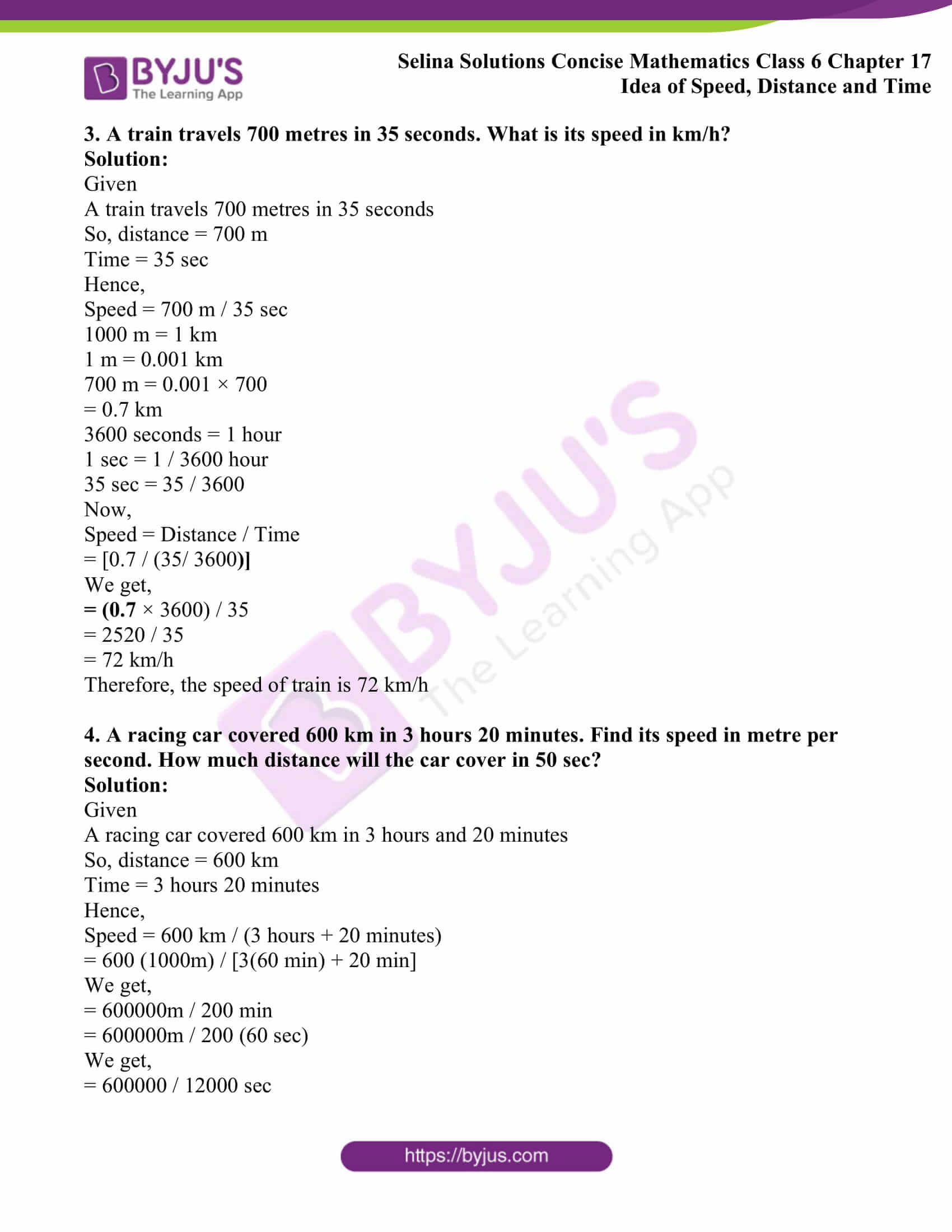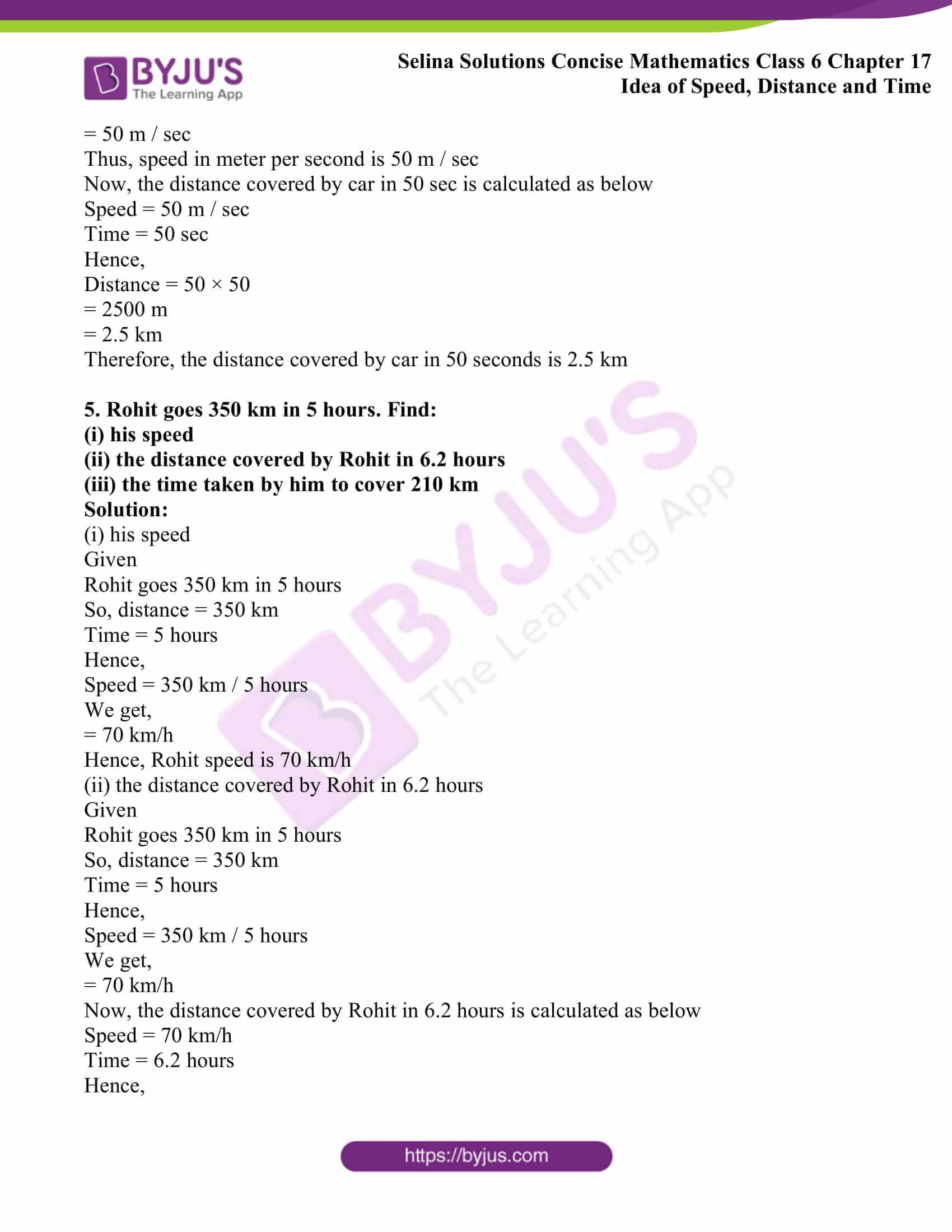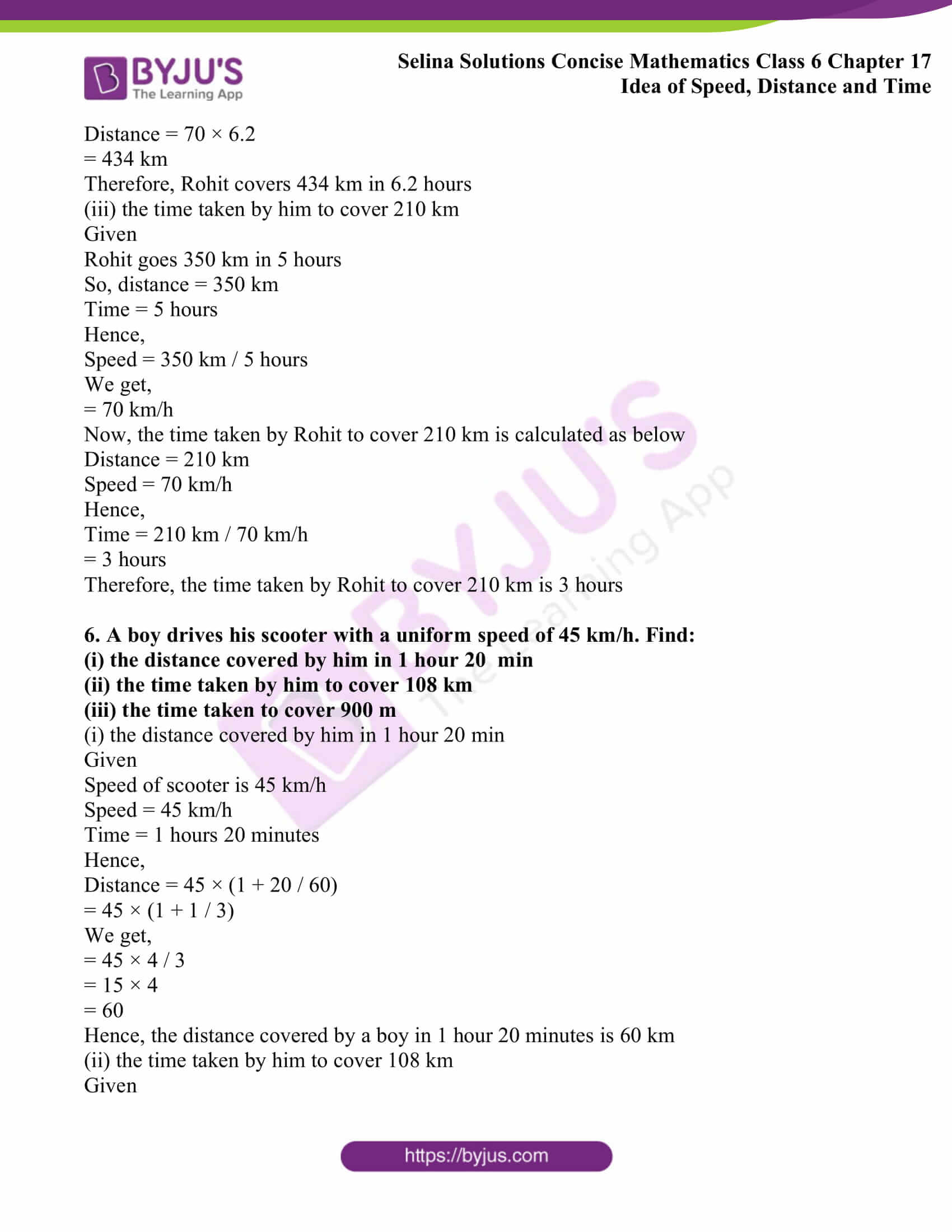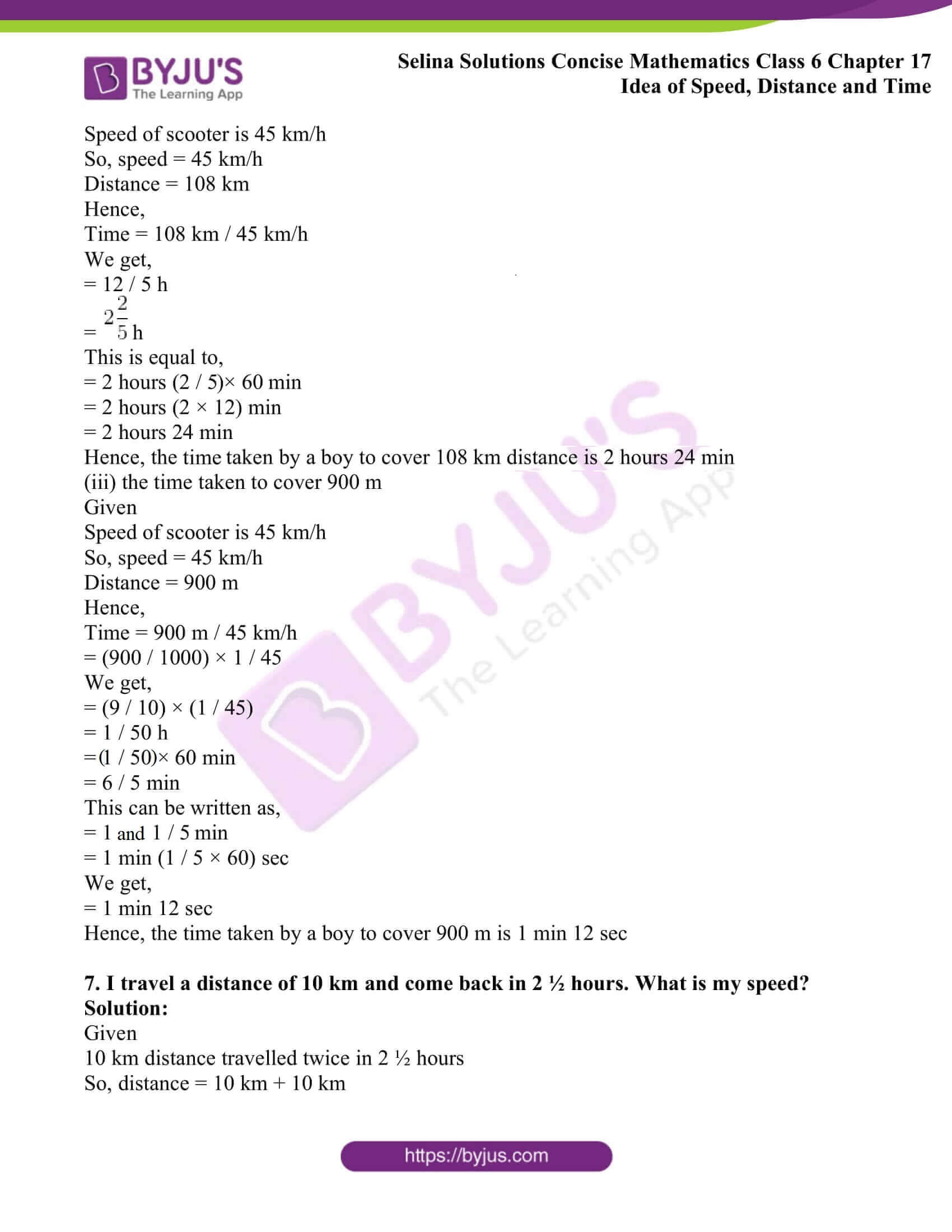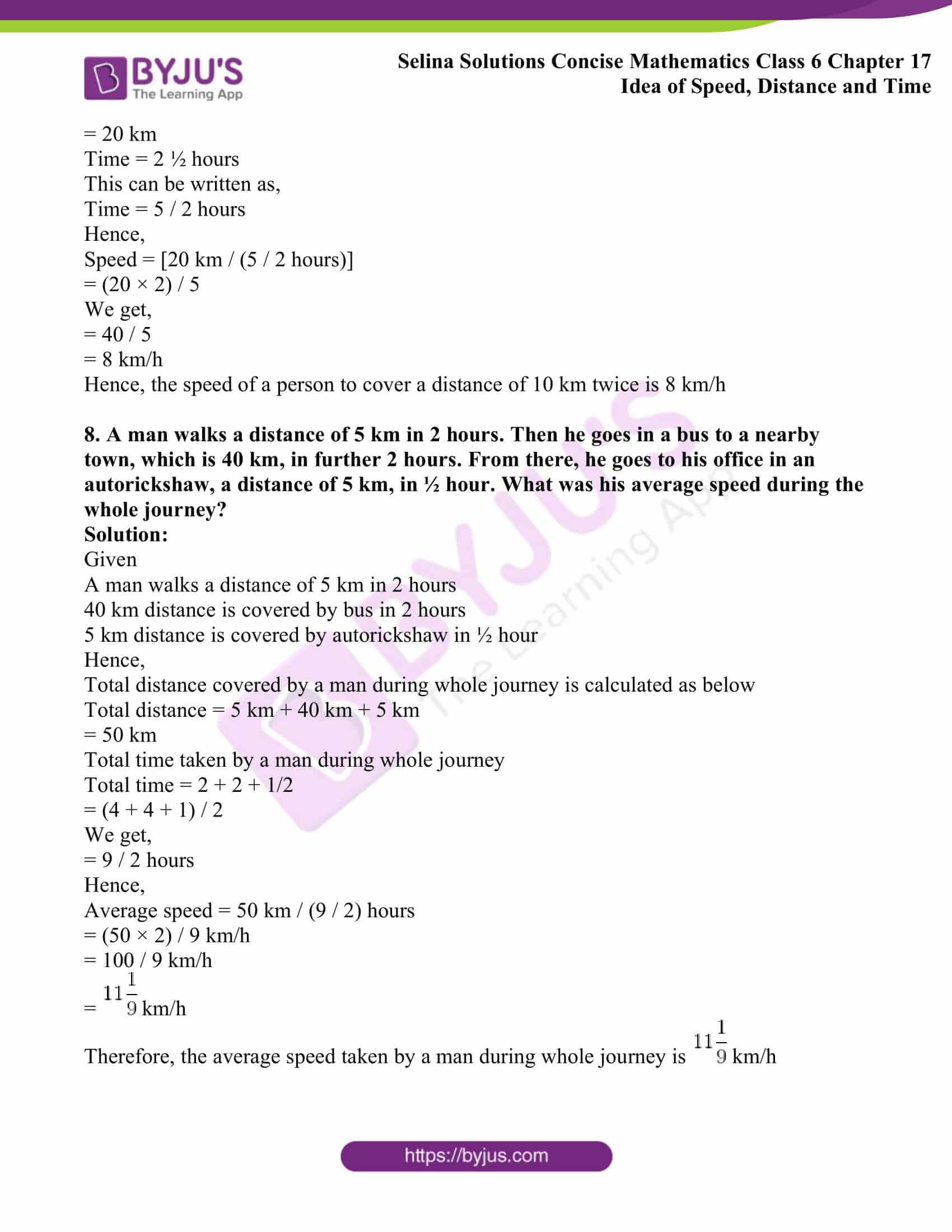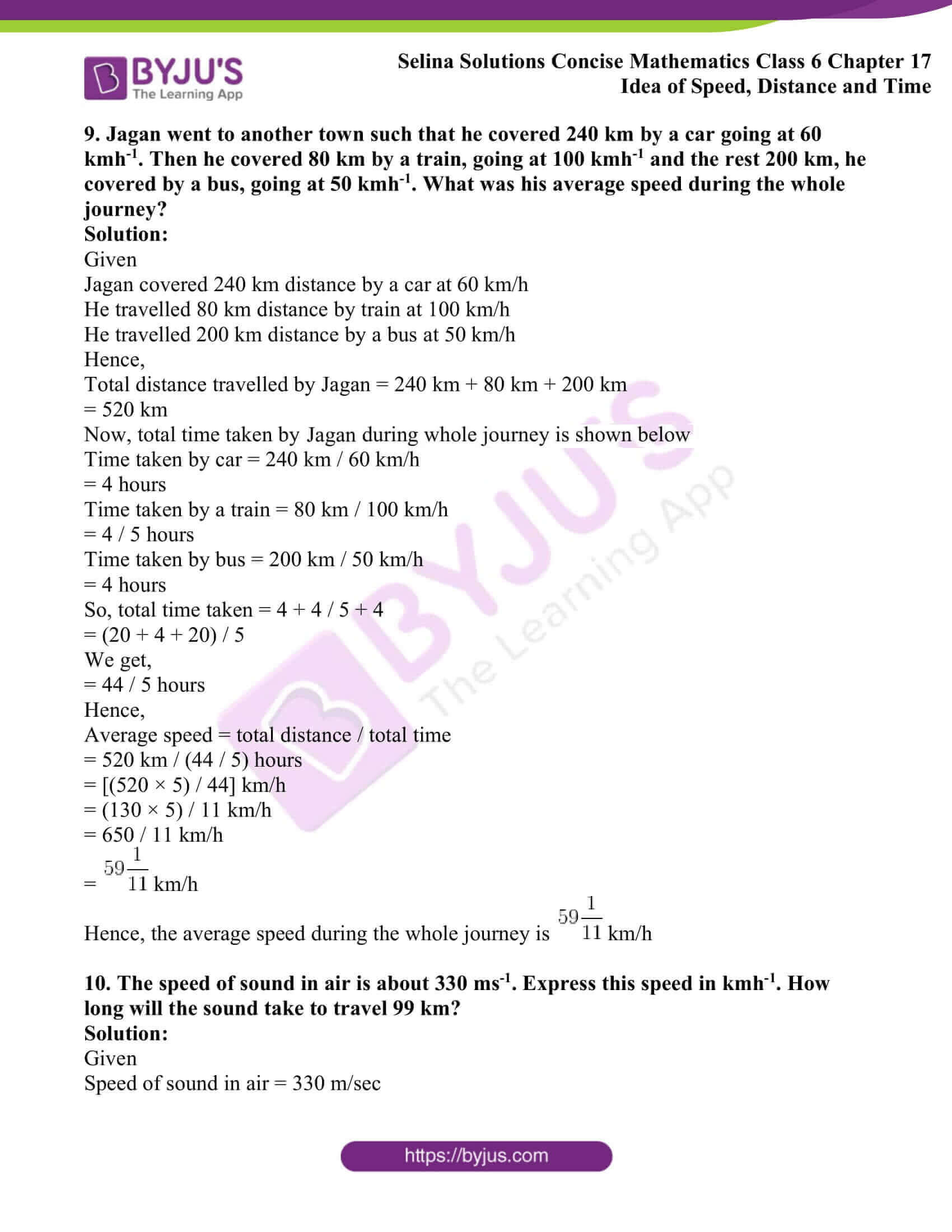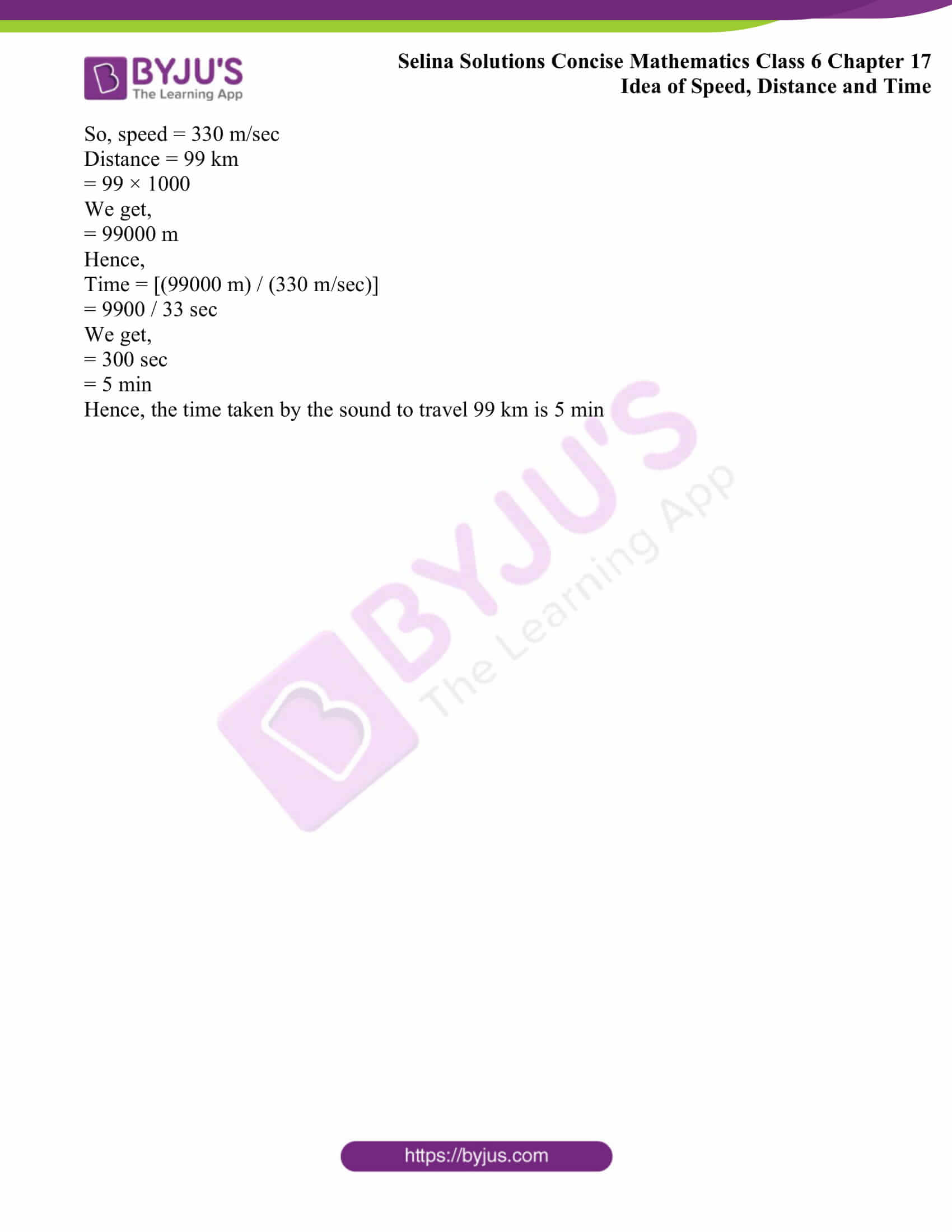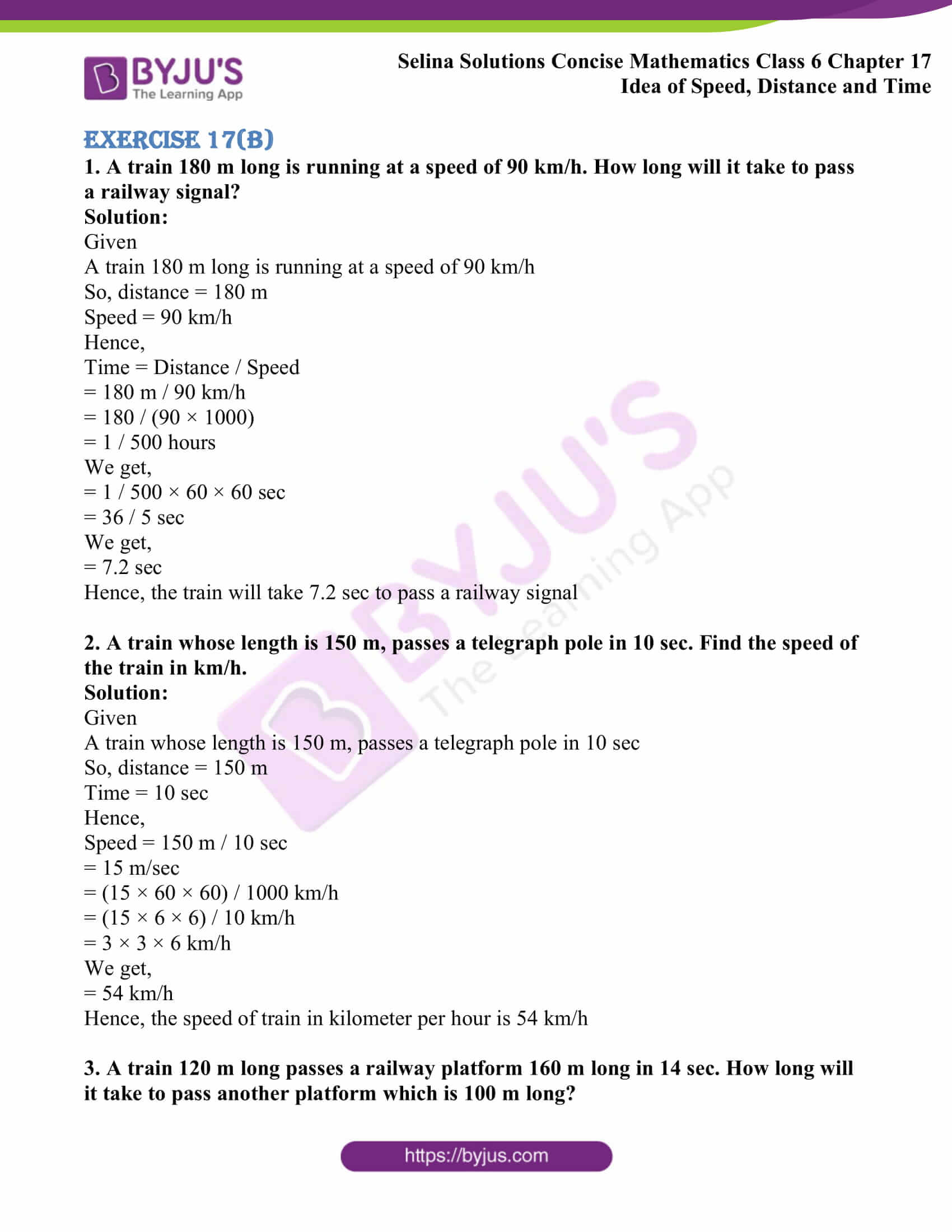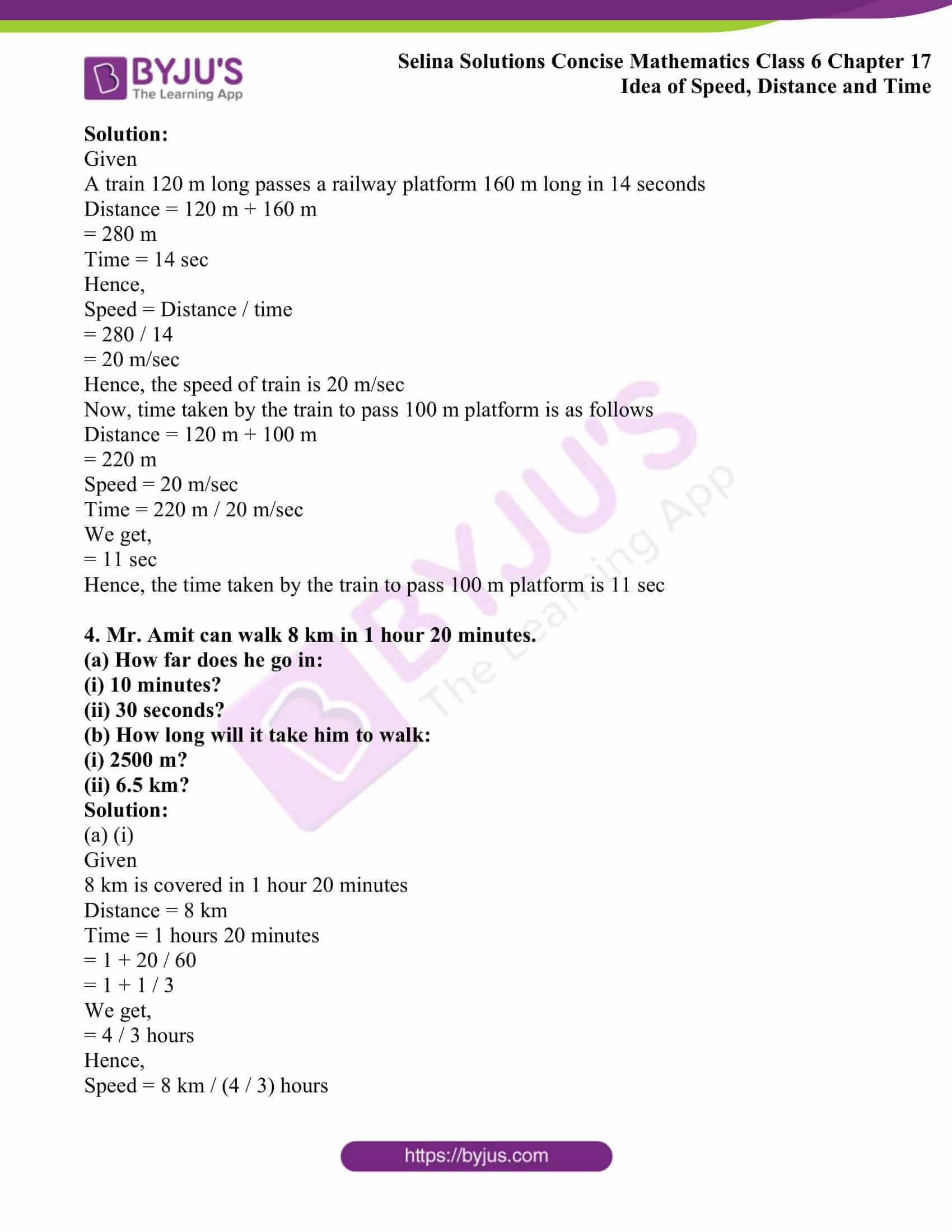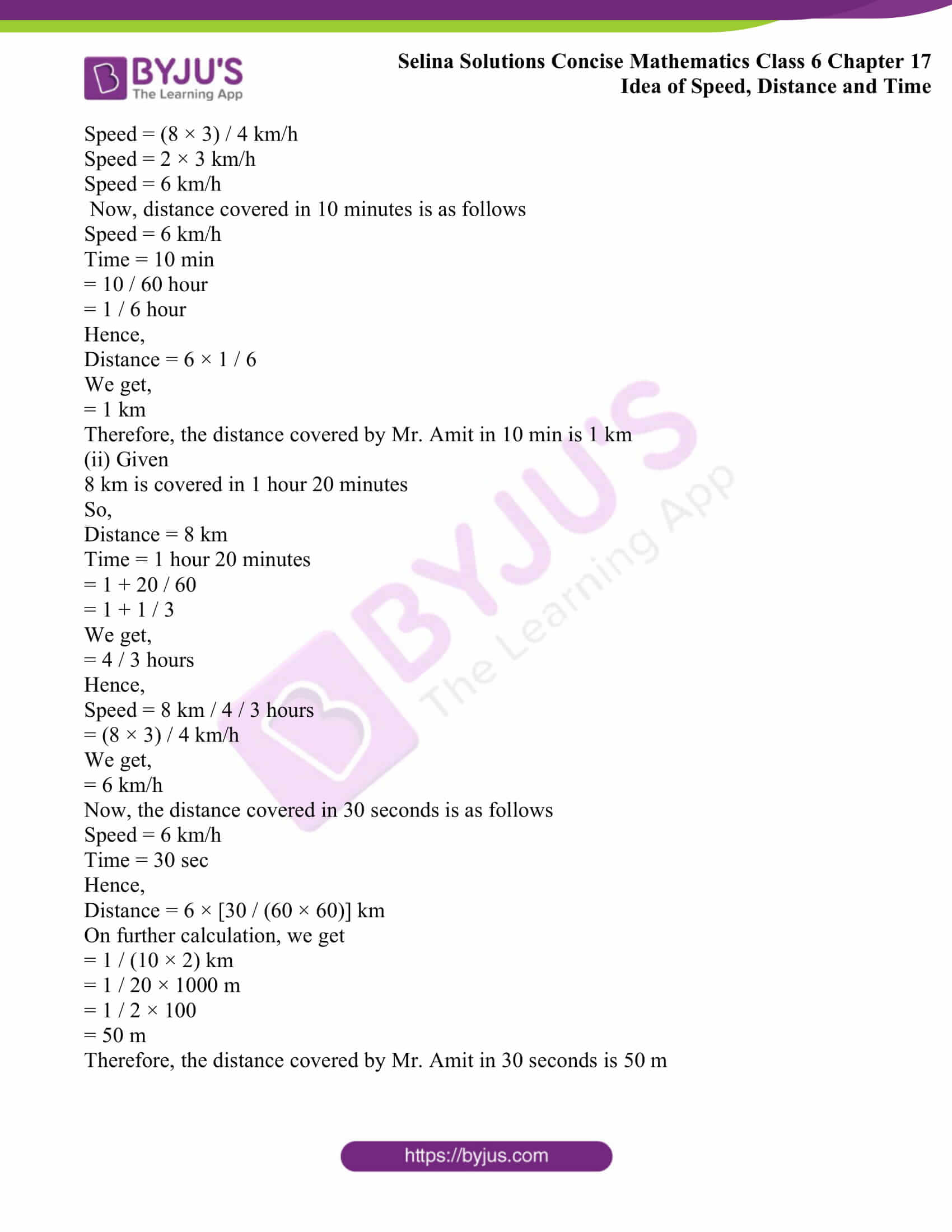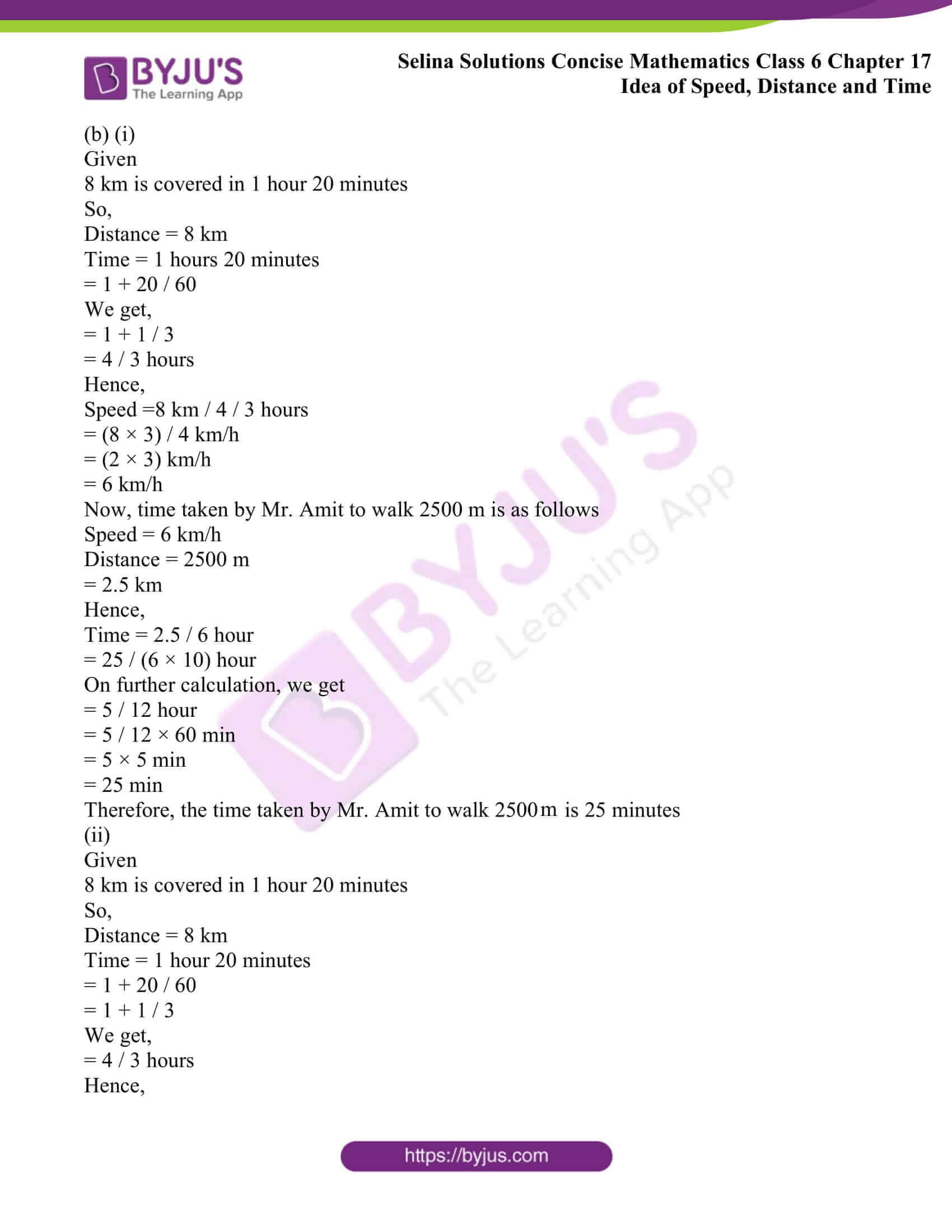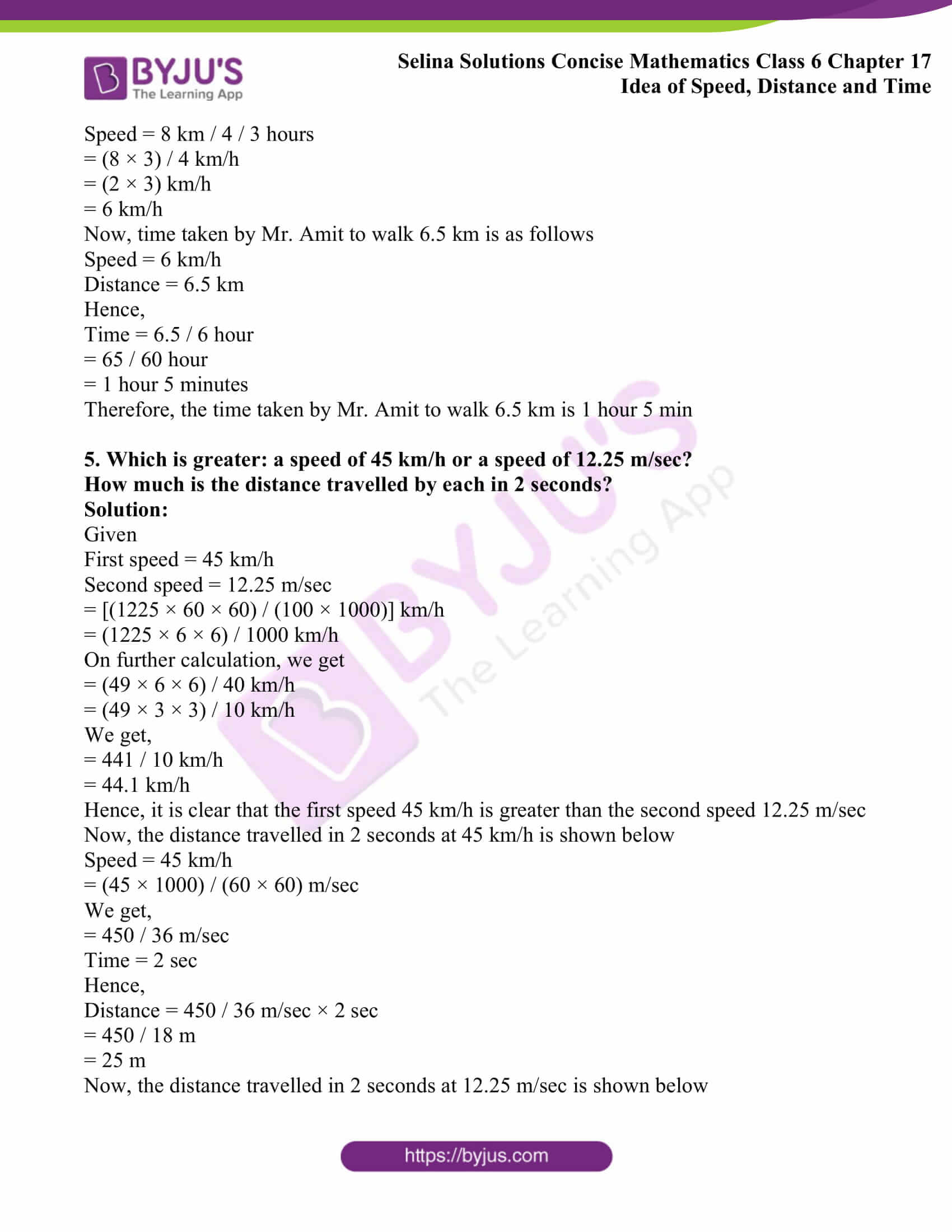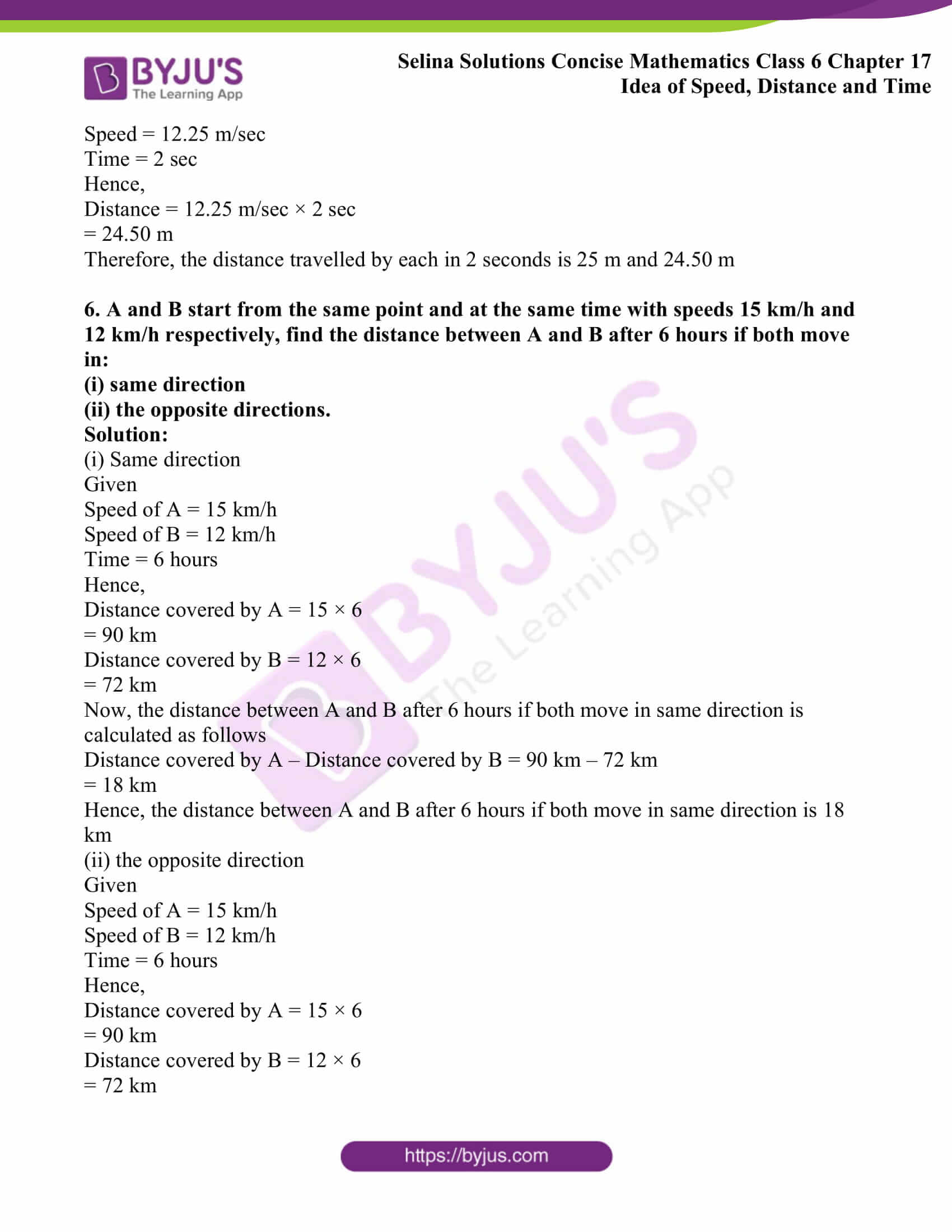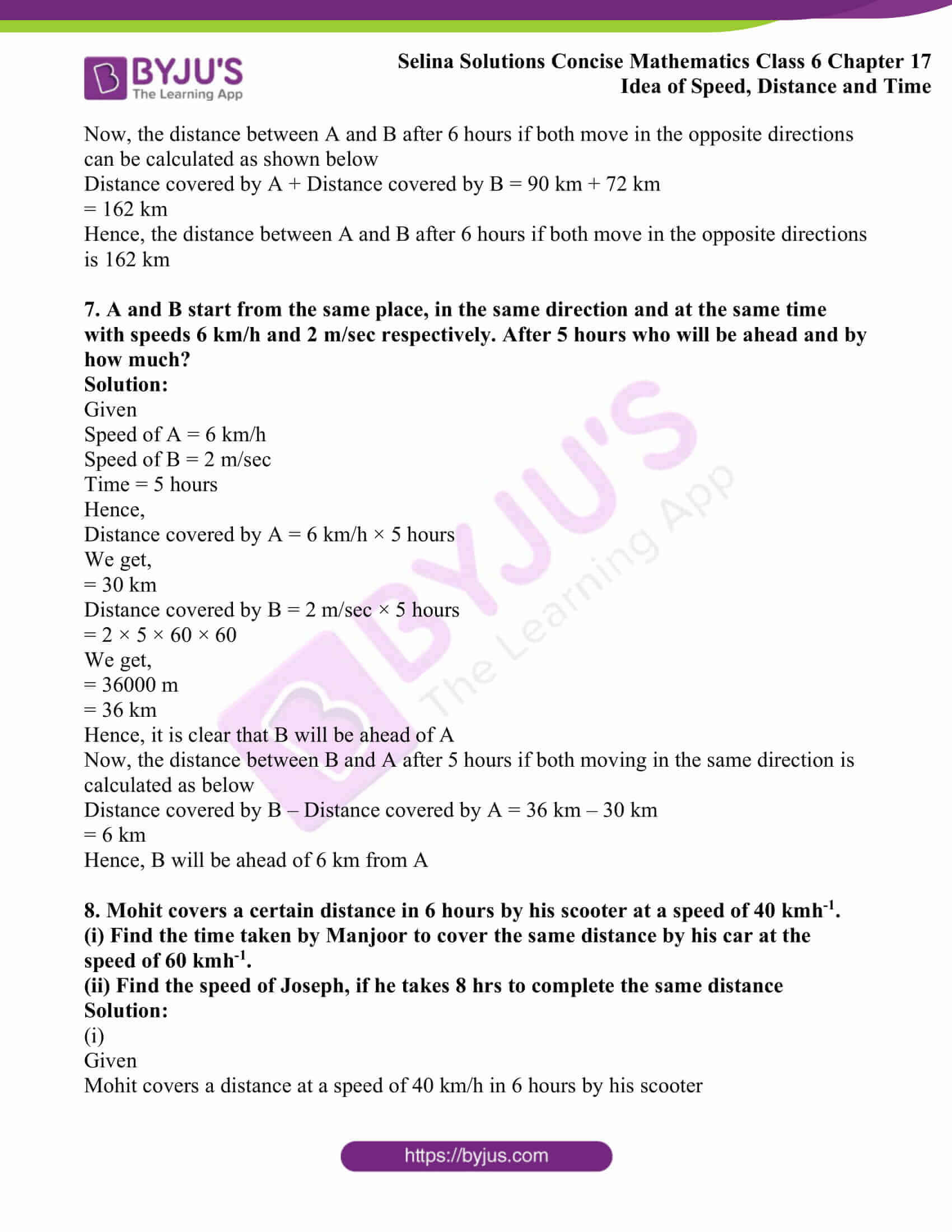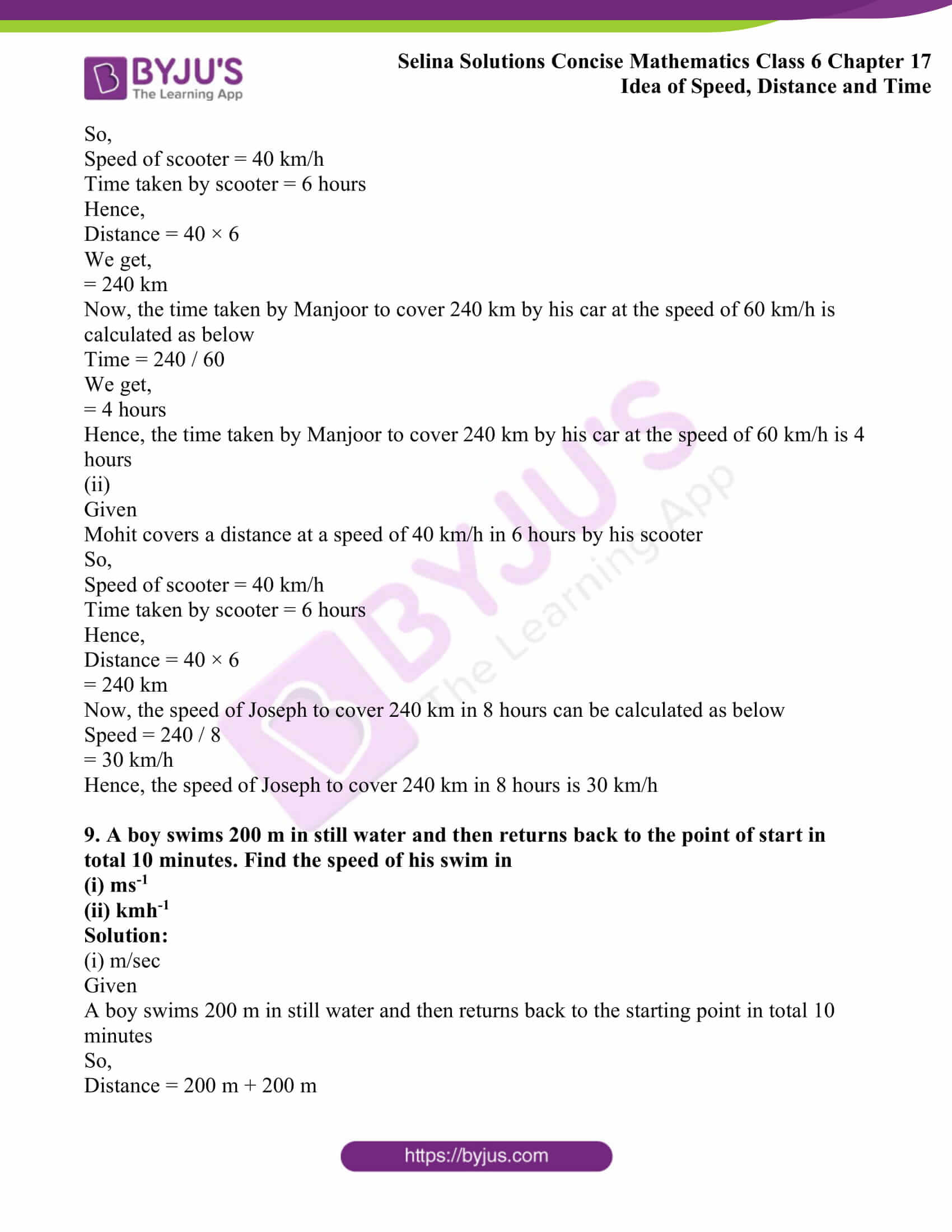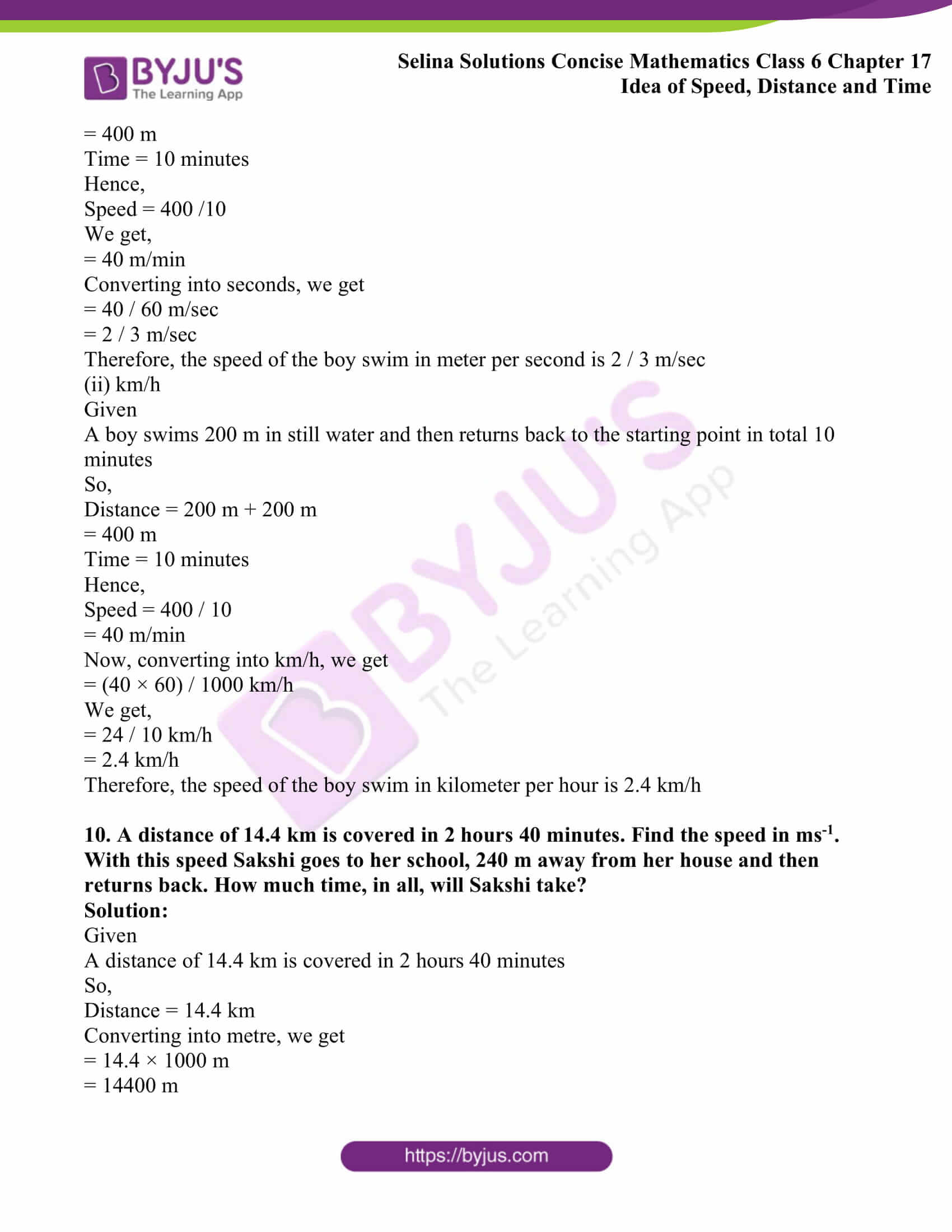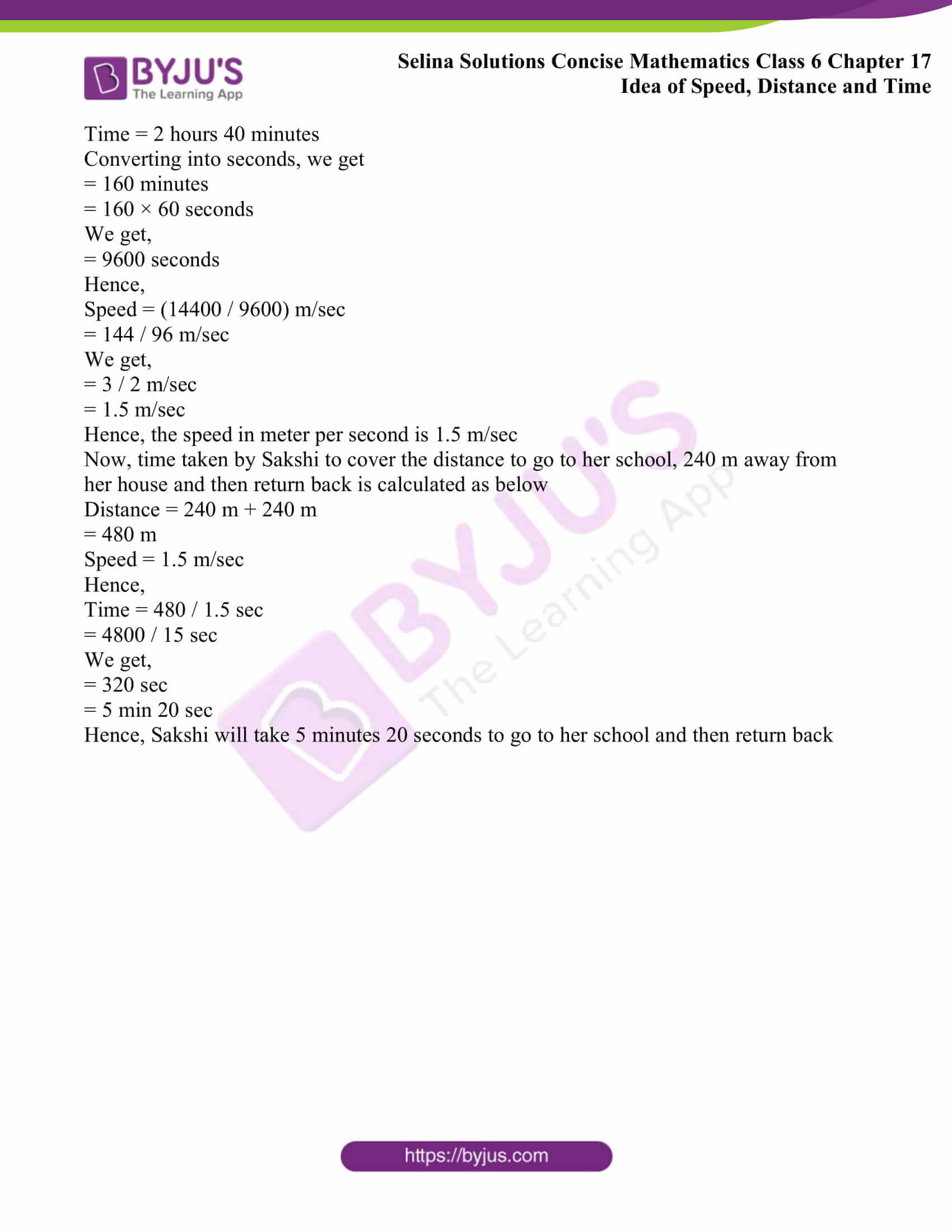### Exercises of Selina Solutions Concise Mathematics Class 6 Chapter 17: Idea of Speed, Distance and Time

Exercise 17(A) Solutions

Exercise 17(B) Solutions

## Access Selina Solutions Concise Mathematics Class 6 Chapter 17: Idea of Speed, Distance and Time

#### Exercise 17(A)

1. A train covers 51 km in 3 hours. Calculate its speed. How far does the train go in 30 minutes?

Solution:

Given

A train covers 51 km in 3 hours

So, distance = 51 km

Time = 3 hours

Hence,

Speed = 51 km/ 3 hours

We get,

= 17 km/h

Now for 30 minutes

Speed = 17 km/h

Time = 0.5 hours

Hence,

Distance = 17 × 0.5

We get,

= 8.5 km

Therefore, train covered 8.5 km in 30 minutes

2. A motorist travelled the distance between two towns, which is 65 km, in 2 hours and 10 minutes. Find his speed in metre per minute.

Solution:

Given

A motorist travelled the distance 65 km in 2 hours and 10 minutes

So, distance = 65 km

Time = 2 hours 10 minutes

Hence,

Speed = 65 km / (2 hours + 10 minutes)

We know that,

1 km = 1000 m

1 hour = 60 min

= 65 (1000 m) / [2 (60 min) + 10 min]

= 65000 m/130 min

We get,

= 500 m/min

Therefore, the speed of a motorist in meter per minute is 500 m/min

3. A train travels 700 metres in 35 seconds. What is its speed in km/h?

Solution:

Given

A train travels 700 metres in 35 seconds

So, distance = 700 m

Time = 35 sec

Hence,

Speed = 700 m / 35 sec

1000 m = 1 km

1 m = 0.001 km

700 m = 0.001 × 700

= 0.7 km

3600 seconds = 1 hour

1 sec = 1 / 3600 hour

35 sec = 35 / 3600

Now,

Speed = Distance / Time

= [0.7 / (35/ 3600)]

We get,

= (0.7 × 3600) / 35

= 2520 / 35

= 72 km/h

Therefore, the speed of train is 72 km/h

4. A racing car covered 600 km in 3 hours 20 minutes. Find its speed in metre per second. How much distance will the car cover in 50 sec?

Solution:

Given

A racing car covered 600 km in 3 hours and 20 minutes

So, distance = 600 km

Time = 3 hours 20 minutes

Hence,

Speed = 600 km / (3 hours + 20 minutes)

= 600 (1000m) / [3(60 min) + 20 min]

We get,

= 600000m / 200 min

= 600000m / 200 (60 sec)

We get,

= 600000 / 12000 sec

= 50 m / sec

Thus, speed in meter per second is 50 m / sec

Now, the distance covered by car in 50 sec is calculated as below

Speed = 50 m / sec

Time = 50 sec

Hence,

Distance = 50 × 50

= 2500 m

= 2.5 km

Therefore, the distance covered by car in 50 seconds is 2.5 km

5. Rohit goes 350 km in 5 hours. Find:

(i) his speed

(ii) the distance covered by Rohit in 6.2 hours

(iii) the time taken by him to cover 210 km

Solution:

(i) his speed

Given

Rohit goes 350 km in 5 hours

So, distance = 350 km

Time = 5 hours

Hence,

Speed = 350 km / 5 hours

We get,

= 70 km/h

Hence, Rohit speed is 70 km/h

(ii) the distance covered by Rohit in 6.2 hours

Given

Rohit goes 350 km in 5 hours

So, distance = 350 km

Time = 5 hours

Hence,

Speed = 350 km / 5 hours

We get,

= 70 km/h

Now, the distance covered by Rohit in 6.2 hours is calculated as below

Speed = 70 km/h

Time = 6.2 hours

Hence,

Distance = 70 × 6.2

= 434 km

Therefore, Rohit covers 434 km in 6.2 hours

(iii) the time taken by him to cover 210 km

Given

Rohit goes 350 km in 5 hours

So, distance = 350 km

Time = 5 hours

Hence,

Speed = 350 km / 5 hours

We get,

= 70 km/h

Now, the time taken by Rohit to cover 210 km is calculated as below

Distance = 210 km

Speed = 70 km/h

Hence,

Time = 210 km / 70 km/h

= 3 hours

Therefore, the time taken by Rohit to cover 210 km is 3 hours

6. A boy drives his scooter with a uniform speed of 45 km/h. Find:

(i) the distance covered by him in 1 hour 20 min

(ii) the time taken by him to cover 108 km

(iii) the time taken to cover 900 m

(i) the distance covered by him in 1 hour 20 min

Given

Speed of scooter is 45 km/h

Speed = 45 km/h

Time = 1 hours 20 minutes

Hence,

Distance = 45 × (1 + 20 / 60)

= 45 × (1 + 1 / 3)

We get,

= 45 × 4 / 3

= 15 × 4

= 60

Hence, the distance covered by a boy in 1 hour 20 minutes is 60 km

(ii) the time taken by him to cover 108 km

Given

Speed of scooter is 45 km/h

So, speed = 45 km/h

Distance = 108 km

Hence,

Time = 108 km / 45 km/h

We get,

= 12 / 5 h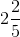h

This is equal to,

= 2 hours (2 / 5) × 60 min

= 2 hours (2 × 12) min

= 2 hours 24 min

Hence, the time taken by a boy to cover 108 km distance is 2 hours 24 min

(iii) the time taken to cover 900 m

Given

Speed of scooter is 45 km/h

So, speed = 45 km/h

Distance = 900 m

Hence,

Time = 900 m / 45 km/h

= (900 / 1000) × 1 / 45

We get,

= (9 / 10) × (1 / 45)

= 1 / 50 h

= (1 / 50) × 60 min

= 6 / 5 min

This can be written as,

= 1 and 1 / 5 min

= 1 min (1 / 5 × 60) sec

We get,

= 1 min 12 sec

Hence, the time taken by a boy to cover 900 m is 1 min 12 sec

7. I travel a distance of 10 km and come back in 2 ½ hours. What is my speed?

Solution:

Given

10 km distance travelled twice in 2 ½ hours

So, distance = 10 km + 10 km

= 20 km

Time = 2 ½ hours

This can be written as,

Time = 5 / 2 hours

Hence,

Speed = [20 km / (5 / 2 hours)]

= (20 × 2) / 5

We get,

= 40 / 5

= 8 km/h

Hence, the speed of a person to cover a distance of 10 km twice is 8 km/h

8. A man walks a distance of 5 km in 2 hours. Then he goes in a bus to a nearby town, which is 40 km, in further 2 hours. From there, he goes to his office in an autorickshaw, a distance of 5 km, in ½ hour. What was his average speed during the whole journey?

Solution:

Given

A man walks a distance of 5 km in 2 hours

40 km distance is covered by bus in 2 hours

5 km distance is covered by autorickshaw in ½ hour

Hence,

Total distance covered by a man during whole journey is calculated as below

Total distance = 5 km + 40 km + 5 km

= 50 km

Total time taken by a man during whole journey

Total time = 2 + 2 + 1/2

= (4 + 4 + 1) / 2

We get,

= 9 / 2 hours

Hence,

Average speed = 50 km / (9 / 2) hours

= (50 × 2) / 9 km/h

= 100 / 9 km/h

=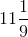km/h

Therefore, the average speed taken by a man during whole journey iskm/h

9. Jagan went to another town such that he covered 240 km by a car going at 60 kmh-1. Then he covered 80 km by a train, going at 100 kmh-1 and the rest 200 km, he covered by a bus, going at 50 kmh-1. What was his average speed during the whole journey?

Solution:

Given

Jagan covered 240 km distance by a car at 60 km/h

He travelled 80 km distance by train at 100 km/h

He travelled 200 km distance by a bus at 50 km/h

Hence,

Total distance travelled by Jagan = 240 km + 80 km + 200 km

= 520 km

Now, total time taken by Jagan during whole journey is shown below

Time taken by car = 240 km / 60 km/h

= 4 hours

Time taken by a train = 80 km / 100 km/h

= 4 / 5 hours

Time taken by bus = 200 km / 50 km/h

= 4 hours

So, total time taken = 4 + 4 / 5 + 4

= (20 + 4 + 20) / 5

We get,

= 44 / 5 hours

Hence,

Average speed = total distance / total time

= 520 km / (44 / 5) hours

= [(520 × 5) / 44] km/h

= (130 × 5) / 11 km/h

= 650 / 11 km/h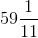km/h

Hence, the average speed during the whole journey iskm/h

10. The speed of sound in air is about 330 ms-1. Express this speed in kmh-1. How long will the sound take to travel 99 km?

Solution:

Given

Speed of sound in air = 330 m/sec

So, speed = 330 m/sec

Distance = 99 km

= 99 × 1000

We get,

= 99000 m

Hence,

Time = [(99000 m) / (330 m/sec)]

= 9900 / 33 sec

We get,

= 300 sec

= 5 min

Hence, the time taken by the sound to travel 99 km is 5 min

#### Exercise 17(B)

1. A train 180 m long is running at a speed of 90 km/h. How long will it take to pass a railway signal?

Solution:

Given

A train 180 m long is running at a speed of 90 km/h

So, distance = 180 m

Speed = 90 km/h

Hence,

Time = Distance / Speed

= 180 m / 90 km/h

= 180 / (90 × 1000)

= 1 / 500 hours

We get,

= 1 / 500 × 60 × 60 sec

= 36 / 5 sec

We get,

= 7.2 sec

Hence, the train will take 7.2 sec to pass a railway signal

2. A train whose length is 150 m, passes a telegraph pole in 10 sec. Find the speed of the train in km/h.

Solution:

Given

A train whose length is 150 m, passes a telegraph pole in 10 sec

So, distance = 150 m

Time = 10 sec

Hence,

Speed = 150 m / 10 sec

= 15 m/sec

= (15 × 60 × 60) / 1000 km/h

= (15 × 6 × 6) / 10 km/h

= 3 × 3 × 6 km/h

We get,

= 54 km/h

Hence, the speed of train in kilometer per hour is 54 km/h

3. A train 120 m long passes a railway platform 160 m long in 14 sec. How long will it take to pass another platform which is 100 m long?

Solution:

Given

A train 120 m long passes a railway platform 160 m long in 14 seconds

Distance = 120 m + 160 m

= 280 m

Time = 14 sec

Hence,

Speed = Distance / time

= 280 / 14

= 20 m/sec

Hence, the speed of train is 20 m/sec

Now, time taken by the train to pass 100 m platform is as follows

Distance = 120 m + 100 m

= 220 m

Speed = 20 m/sec

Time = 220 m / 20 m/sec

We get,

= 11 sec

Hence, the time taken by the train to pass 100 m platform is 11 sec

4. Mr. Amit can walk 8 km in 1 hour 20 minutes.

(a) How far does he go in:

(i) 10 minutes?

(ii) 30 seconds?

(b) How long will it take him to walk:

(i) 2500 m?

(ii) 6.5 km?

Solution:

(a) (i)

Given

8 km is covered in 1 hour 20 minutes

Distance = 8 km

Time = 1 hour 20 minutes

= 1 + 20 / 60

= 1 + 1 / 3

We get,

= 4 / 3 hours

Hence,

Speed = 8 km / (4 / 3) hours

Speed = (8 × 3) / 4 km/h

Speed = 2 × 3 km/h

Speed = 6 km/h

Now, distance covered in 10 minutes is as follows

Speed = 6 km/h

Time = 10 min

= 10 / 60 hour

= 1 / 6 hour

Hence,

Distance = 6 × 1 / 6

We get,

= 1 km

Therefore, the distance covered by Mr. Amit in 10 min is 1 km

(ii) Given

8 km is covered in 1 hour 20 minutes

So,

Distance = 8 km

Time = 1 hour 20 minutes

= 1 + 20 / 60

= 1 + 1 / 3

We get,

= 4 / 3 hours

Hence,

Speed = 8 km / 4 / 3 hours

= (8 × 3) / 4 km/h

We get,

= 6 km/h

Now, the distance covered in 30 seconds is as follows

Speed = 6 km/h

Time = 30 sec

Hence,

Distance = 6 × [30 / (60 × 60)] km

On further calculation, we get

= 1 / (10 × 2) km

= 1 / 20 × 1000 m

= 1 / 2 × 100

= 50 m

Therefore, the distance covered by Mr. Amit in 30 seconds is 50 m

(b) (i)

Given

8 km is covered in 1 hour 20 minutes

So,

Distance = 8 km

Time = 1 hour 20 minutes

= 1 + 20 / 60

We get,

= 1 + 1 / 3

= 4 / 3 hours

Hence,

Speed = 8 km / 4 / 3 hours

= (8 × 3) / 4 km/h

= (2 × 3) km/h

= 6 km/h

Now, time taken by Mr. Amit to walk 2500 m is as follows

Speed = 6 km/h

Distance = 2500 m

= 2.5 km

Hence,

Time = 2.5 / 6 hour

= 25 / (6 × 10) hour

On further calculation, we get

= 5 / 12 hour

= 5 / 12 × 60 min

= 5 × 5 min

= 25 min

Therefore, the time taken by Mr. Amit to walk 2500 m is 25 minutes

(ii)

Given

8 km is covered in 1 hour 20 minutes

So,

Distance = 8 km

Time = 1 hour 20 minutes

= 1 + 20 / 60

= 1 + 1 / 3

We get,

= 4 / 3 hours

Hence,

Speed = 8 km / 4 / 3 hours

= (8 × 3) / 4 km/h

= (2 × 3) km/h

= 6 km/h

Now, time taken by Mr. Amit to walk 6.5 km is as follows

Speed = 6 km/h

Distance = 6.5 km

Hence,

Time = 6.5 / 6 hour

= 65 / 60 hour

= 1 hour 5 minutes

Therefore, the time taken by Mr. Amit to walk 6.5 km is 1 hour 5 min

5. Which is greater: a speed of 45 km/h or a speed of 12.25 m/sec?

How much is the distance travelled by each in 2 seconds?

Solution:

Given

First speed = 45 km/h

Second speed = 12.25 m/sec

= [(1225 × 60 × 60) / (100 × 1000)] km/h

= (1225 × 6 × 6) / 1000 km/h

On further calculation, we get

= (49 × 6 × 6) / 40 km/h

= (49 × 3 × 3) / 10 km/h

We get,

= 441 / 10 km/h

= 44.1 km/h

Hence, it is clear that the first speed 45 km/h is greater than the second speed 12.25 m/sec

Now, the distance travelled in 2 seconds at 45 km/h is shown below

Speed = 45 km/h

= (45 × 1000) / (60 × 60) m/sec

We get,

= 450 / 36 m/sec

Time = 2 sec

Hence,

Distance = 450 / 36 m/sec × 2 sec

= 450 / 18 m

= 25 m

Now, the distance travelled in 2 seconds at 12.25 m/sec is shown below

Speed = 12.25 m/sec

Time = 2 sec

Hence,

Distance = 12.25 m/sec × 2 sec

= 24.50 m

Therefore, the distance travelled by each in 2 seconds is 25 m and 24.50 m

6. A and B start from the same point and at the same time with speeds 15 km/h and 12 km/h respectively, find the distance between A and B after 6 hours if both move in:

(i) same direction

(ii) the opposite directions.

Solution:

(i) Same direction

Given

Speed of A = 15 km/h

Speed of B = 12 km/h

Time = 6 hours

Hence,

Distance covered by A = 15 × 6

= 90 km

Distance covered by B = 12 × 6

= 72 km

Now, the distance between A and B after 6 hours if both move in same direction is calculated as follows

Distance covered by A – Distance covered by B = 90 km – 72 km

= 18 km

Hence, the distance between A and B after 6 hours if both move in same direction is 18 km

(ii) the opposite direction

Given

Speed of A = 15 km/h

Speed of B = 12 km/h

Time = 6 hours

Hence,

Distance covered by A = 15 × 6

= 90 km

Distance covered by B = 12 × 6

= 72 km

Now, the distance between A and B after 6 hours if both move in the opposite directions can be calculated as shown below

Distance covered by A + Distance covered by B = 90 km + 72 km

= 162 km

Hence, the distance between A and B after 6 hours if both move in the opposite directions is 162 km

7. A and B start from the same place, in the same direction and at the same time with speeds 6 km/h and 2 m/sec respectively. After 5 hours who will be ahead and by how much?

Solution:

Given

Speed of A = 6 km/h

Speed of B = 2 m/sec

Time = 5 hours

Hence,

Distance covered by A = 6 km/h × 5 hours

We get,

= 30 km

Distance covered by B = 2 m/sec × 5 hours

= 2 × 5 × 60 × 60

We get,

= 36000 m

= 36 km

Hence, it is clear that B will be ahead of A

Now, the distance between B and A after 5 hours if both moving in the same direction is calculated as below

Distance covered by B – Distance covered by A = 36 km – 30 km

= 6 km

Hence, B will be ahead of 6 km from A

8. Mohit covers a certain distance in 6 hours by his scooter at a speed of 40 kmh-1.

(i) Find the time taken by Manjoor to cover the same distance by his car at the speed of 60 kmh-1.

(ii) Find the speed of Joseph, if he takes 8 hrs to complete the same distance

Solution:

(i)

Given

Mohit covers a distance at a speed of 40 km/h in 6 hours by his scooter

So,

Speed of scooter = 40 km/h

Time taken by scooter = 6 hours

Hence,

Distance = 40 × 6

We get,

= 240 km

Now, the time taken by Manjoor to cover 240 km by his car at the speed of 60 km/h is calculated as below

Time = 240 / 60

We get,

= 4 hours

Hence, the time taken by Manjoor to cover 240 km by his car at the speed of 60 km/h is 4 hours

(ii)

Given

Mohit covers a distance at a speed of 40 km/h in 6 hours by his scooter

So,

Speed of scooter = 40 km/h

Time taken by scooter = 6 hours

Hence,

Distance = 40 × 6

= 240 km

Now, the speed of Joseph to cover 240 km in 8 hours can be calculated as below

Speed = 240 / 8

= 30 km/h

Hence, the speed of Joseph to cover 240 km in 8 hours is 30 km/h

9. A boy swims 200 m in still water and then returns back to the point of start in total 10 minutes. Find the speed of his swim in

(i) ms-1

(ii) kmh-1

Solution:

(i) m/sec

Given

A boy swims 200 m in still water and then returns back to the starting point in total 10 minutes

So,

Distance = 200 m + 200 m

= 400 m

Time = 10 minutes

Hence,

Speed = 400 /10

We get,

= 40 m/min

Converting into seconds, we get

= 40 / 60 m/sec

= 2 / 3 m/sec

Therefore, the speed of the boy swim in meter per second is 2 / 3 m/sec

(ii) km/h

Given

A boy swims 200 m in still water and then returns back to the starting point in total 10 minutes

So,

Distance = 200 m + 200 m

= 400 m

Time = 10 minutes

Hence,

Speed = 400 / 10

= 40 m/min

Now, converting into km/h, we get

= (40 × 60) / 1000 km/h

We get,

= 24 / 10 km/h

= 2.4 km/h

Therefore, the speed of the boy swim in kilometer per hour is 2.4 km/h

10. A distance of 14.4 km is covered in 2 hours 40 minutes. Find the speed in ms-1. With this speed Sakshi goes to her school, 240 m away from her house and then returns back. How much time, in all, will Sakshi take?

Solution:

Given

A distance of 14.4 km is covered in 2 hours 40 minutes

So,

Distance = 14.4 km

Converting into metre, we get

= 14.4 × 1000 m

= 14400 m

Time = 2 hours 40 minutes

Converting into seconds, we get

= 160 minutes

= 160 × 60 seconds

We get,

= 9600 seconds

Hence,

Speed = (14400 / 9600) m/sec

= 144 / 96 m/sec

We get,

= 3 / 2 m/sec

= 1.5 m/sec

Hence, the speed in meter per second is 1.5 m/sec

Now, time taken by Sakshi to cover the distance to go to her school, 240 m away from her house and then return back is calculated as below

Distance = 240 m + 240 m

= 480 m

Speed = 1.5 m/sec

Hence,

Time = 480 / 1.5 sec

= 4800 / 15 sec

We get,

= 320 sec

= 5 min 20 sec

Hence, Sakshi will take 5 minutes 20 seconds to go to her school and then return back